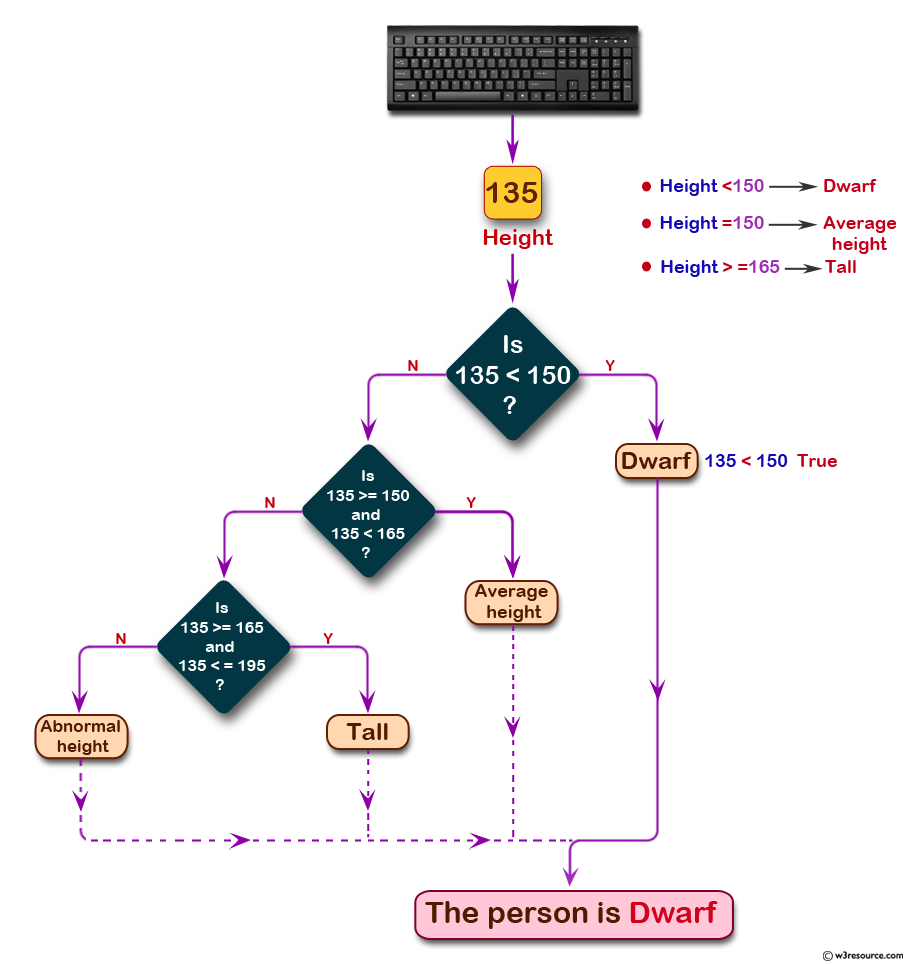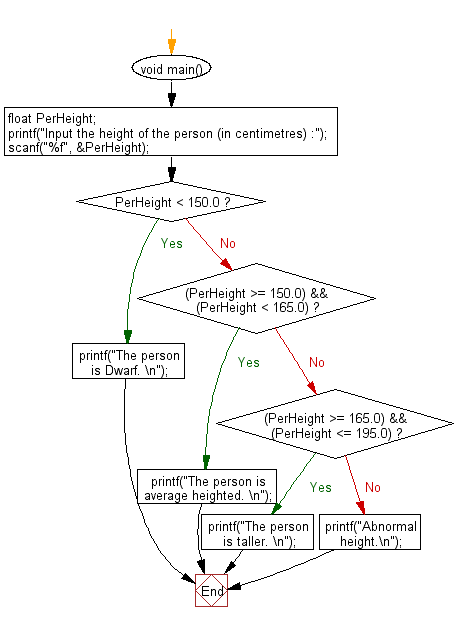﻿ C Program: In centimeters, categorize a person's height# C Exercises: Accept the height of a person in centimeter and categorize them

## C Conditional Statement: Exercise-7 with Solution

Write a C program to accept the height of a person in centimeter and categorize the person according to their height.

Pictorial Presentation:Sample Solution:

C Code:

``````#include <stdio.h>
void main()
{
float PerHeight;

printf("Input the height of the person (in centimetres) :");
scanf("%f", &PerHeight);
if (PerHeight < 150.0)
printf("The person is Dwarf. \n");
else if ((PerHeight >= 150.0) && (PerHeight < 165.0))
printf("The person is  average heighted. \n");
else if ((PerHeight >= 165.0) && (PerHeight <= 195.0))
printf("The person is taller. \n");
else
printf("Abnormal height.\n");
}
```
```

Sample Output:

```Input the height of the person (in centimetres) :135
The person is Dwarf.
```

Flowchart:C Programming Code Editor:

Improve this sample solution and post your code through Disqus.

What is the difficulty level of this exercise?

Test your Programming skills with w3resource's quiz.

﻿

## C Programming: Tips of the Day

Why do C and C++ compilers allow array lengths in function signatures when they're never enforced?

It is a quirk of the syntax for passing arrays to functions.

Actually it is not possible to pass an array in C. If you write syntax that looks like it should pass the array, what actually happens is that a pointer to the first element of the array is passed instead.

Since the pointer does not include any length information, the contents of your [] in the function formal parameter list are actually ignored.

Ref : https://bit.ly/3fhlvdH